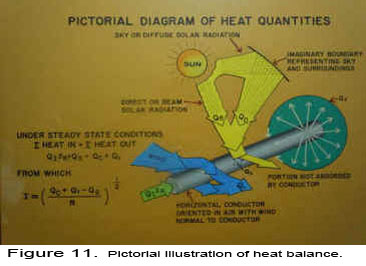# 6. Real Time Thermal Rating

#### Measurements:

The Basic STR Units measure the ambient temperature, solar radiation, conductor temperature, and line current. Also, the Option A Units measure the line sag.

#### Calculations:

According to the heat balance equation under steady state conditions, (I2R) plus the solar radiation received from the sun (Qs) must be equal to the heat lost by thermal radiation (Qr) and convection (Qc), or

I2R+Qs = Qr + Qc.             (1)

Since the STR Unit measures the line current (I) and conductor temperature (tc) of the line, then the (I2R) term is known. Also, the Unit measures the ambient and conductor temperatures, therefore the term Qr is a known quantity. In addition, the Unit measures the total solar radiation Qs, and as a result Qc can then be found directly from equation (1). Furthermore, the surface coefficient of heat transfer h can be determined from Qc, since tc  and ta are measured quantities. It follows that all the values of the terms in equation (1) are obtained directly from the measured values which describe the existing thermal state of the conductor. From this, as shown in Figure 11., the real time value of the current in equation (1) can be found from

I max = ((Qr + Qc – Qs)/R) ½ ,         (2)when the conductor temperature (tc ) is raised to its maximum allowable limit based on loss of tensile strength criteria, which is  tc  versus time dependent and the maximum allowable sag or clearance limit. As will be seen later the Option A STR Units also measure the line sag, therefore the maximum allowable conductor temperature should not be exceeded.

The Real Time Thermal Rating System (RTTRS) of the Powernostics® Software calculates the effective wind speed. During light wind speeds the variability of the wind direction can be quite large. Also there can be heavy gusts of winds during short periods of time. Having an effective wind velocity normal to the conductor is much more useful for calculating the thermal ratings of the overhead line conductors and the de-icing current or ice prevention current.

#### Applications:

1. The Real Time Thermal Rating System ® produces line thermal ratings Imax, through the use of the Powernostics® Software, which are typically 20 to 70% higher than the conventional thermal ratings based on the conservative selection of ambient temperature and wind velocity values. See Figure 12.Normally this conservative selection of ambient weather conditions results in the probability of the wind being lower than the selected value, and the ambient temperature being higher than the selected value to be less than 1 to 2%. Therefore, the calculated steady state thermal line ratings are very conservative.
2. Since conductor temperatures are being measured in real-time, more accurate loss of tensile strength models can be developed and more accurate criteria can be established to prevent exceeding loss of conductor tensile strength limits.
3. Real-time Transient Current Ratings Itr can be found using the Powernostics® Software such as not to exceed safe allowable conductor temperatures based on sag and loss of conductor strength limits. See Figure 13.
4. The critical time (τcrit ) can be calculated for different values of transient currents using the Powernostics® Software to see how long a transient current level can exist without exceeding the cut off conductor temperature (tco). Because the conductor has a thermal time constant, the transient current can far exceed the existing steady state current level.
5.  The Real Time Thermal Rating System ®, based on actual measured conductor temperatures, ambient conditions, and line sags determines what spans within a transmission or distribution line are the critical spans which limits the conductor temperature to the maximum value for the real time rating of the entire line. The Powernostics® Software develops a frequency distribution of these critical spans (fcrit.span), from which the user can identify the location of the most frequently occurring bottlenecks in the line. This information can then be used to determine which spans within the line should be changed out to larger conductor sizes, thus eliminating these bottlenecks and raising the thermal rating value of the line.

#### Benefits:

1. The Real Time Thermal Ratings can be 20 to 70% (see Figure 14.) higher than conventional thermal ratings based on pessimistic selected weather parameters for ambient temperature and wind velocity.
2. Transient Real Time Ratings can be even higher than steady state ratings of which when applied can alleviate opening what is thought to be an overloaded line, when in fact the line is not overloaded at all.
3. The Real TimeThermal Rating System® not only tells the operator when the line rating can be significantly higher than the conservative steady state ratings, but also indicates to the operator when the real time rating is lower than the conservative traditional rating. This condition can occur when the wind velocity is lower than the selected value chosen to calculate the traditional conventional rating, or when the ambient temperature is higher than the selected value. Although this condition is generally less than 1% of the time, parallel wind flows which have magnitudes even higher than the pessimistic assumed perpendicular flow wind can result in much lower convected heat and can cause much higher conductor temperatures than expected. This can cause excessive line sags and power blackouts, which can be avoided with the application of the RTTRS. See Figures 15 and 16.
4. The benefit to cost ratio of just applying the RTTRS often results in values exceeding 25. The installed cost of the STR Unit and Software is orders of magnitude less than re-conductoring an entire line when only certain heavily shielded areas of the line create high frequency of occurrence of critical spans which need to be upgraded, or replaced with larger conductors.